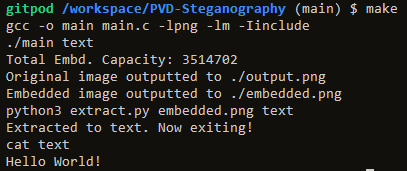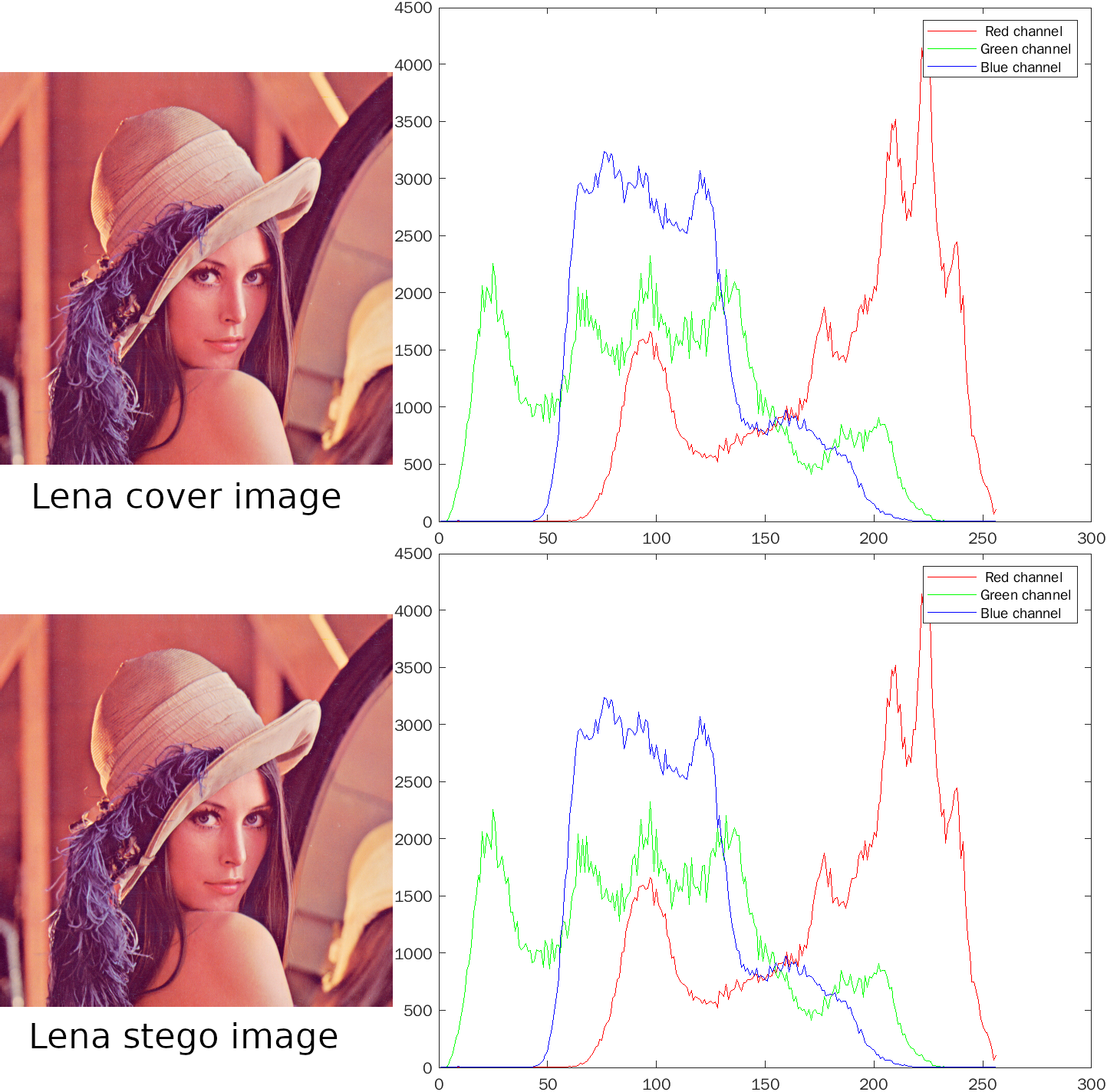[ No 2  ]

## PVD STEGANOGRAPHY

##### Pixel Value Differencing based Steganography (Using LSB)

Programming## Concept

### Basics of pixel-value differencing (PVD)

The PVD method uses grey-level images as the cover image and variable-sized secret message bit sequences are embedded into the cover image. Fewer secret message bit sequences are embedded into the smooth region compared with the edge region.

A cover image is partitioned into non-overlapping blocks of size `1 × 2` in raster scan order. The next steps can be referred from this published paper).

### Application

Instead of grey-level images, a colour image steganographic scheme is proposed. By decomposing each colour pixel into its corresponding colour components, i.e. R, G and B, any small change to one of its components will not affect the overall colour when the pixel is blended in with its surrounding pixels.

In this proposed steganography scheme, bytes, or strings in this case are converted into bits, and then embedded onto the least significant bits (LSB) of pixel RGB components. By using the least significant bits, this bit flip is essentially an absolute difference of 1.

After generating/importing a suitable cover image, the bitmap is partitioned into non-overlapping blocks of `3 x 3` in raster scan order.

Then, per byte of the string we want to embed, randomly choose non-repeating blocks from the previous partition. For each embedded byte, classify its bits to determine whether a smaller sized block is enough to embed them (Whether it is `2 x 2` or `3 x 3`).

``````int classify(int pixel_value_difference) {
int nbits = 0;
if (pixel_value_difference < 16) {
nbits = 2;
} else if (pixel_value_difference > 16 && pixel_value_difference < 32) {
nbits = 3;
} else {
nbits = 4;
}
return nbits;
}
``````

These bits are then embedded by bit flipping the least significant bits of chosen colour components of the colour pixels within the partitioned blocks.

``````int embed_bits(...) {
// Classify number of pixels required to embed
int nbits = classify(pixel_value_difference);

// Compare classification and number of bits from string to be embedded
if (nbits < strlen(bits)) {
// Embed on the LSB of chosen colour component
} else {
// Pad chosen colour component to fit number of bits
}
}
``````

The above steps mentioned many random choices, which should not be easily replicated. In this project, I have chosen to log these choices in terms of concatenated bits. This thus makes the steganography system only extractable by those who know the logging system.

``````switch (pixel) {
case 'r':
sequence = '0';
sequence = '0';
break;
case 'g':
sequence = '0';
sequence = '1';
break;
case 'b':
sequence = '1';
sequence = '0';
break;
}

switch (pixel_value_difference) {
case 2:
sequence = '0';
sequence = '0';
break;
case 3:
sequence = '0';
sequence = '1';
break;
case 4:
sequence = '1';
sequence = '0';
break;
}

case 0:
sequence = '0';
sequence = '0';
break;
case 1:
sequence = '0';
sequence = '1';
break;
case 2:
sequence = '1';
sequence = '0';
break;
case 3:
sequence = '1';
sequence = '1';
break;
}

fprintf(logfile_ptr, "%d %d %d \n", i, j, bin_to_char(sequence));
``````

This steganography scheme is symmetrical, so reversing the embedding steps will result back to our original string.

``````# Foreach line in log file

# i, j, sequence according to fprintf from above
i, j, sequence = [int(i) for i in each_line.split()]

# Convert int to bin, pad up to length 7, then split into 3 length-2 blocks
pixel_index, pixel_value_difference_index, padding_index = [i for i in map(''.join, zip(*[iter(bin(sequence)[2:].zfill(7))]*2))]

# Retrieve original configurations based on `00`, `01`, `10` or `11` indexes